• Algorihtm to minimize the cost function(BA) in particular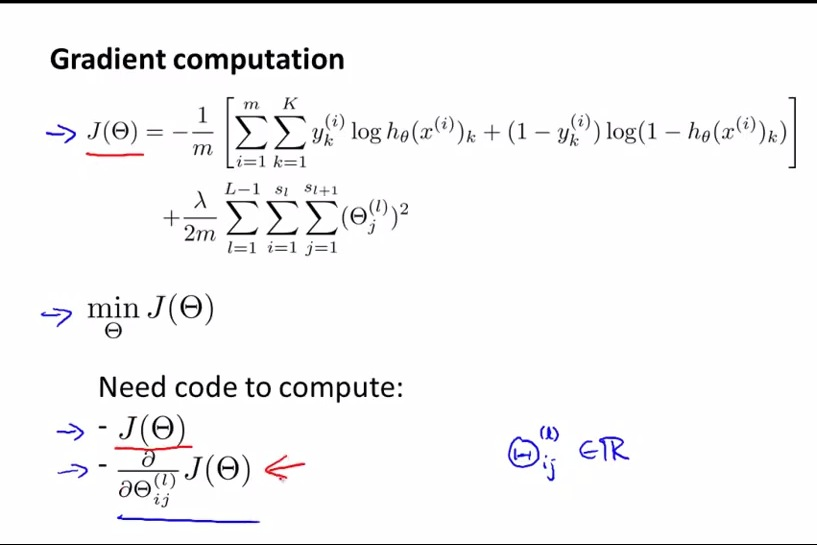• Need to compute the partial derrivative
• Keep in mind that the hyphothesis is the row number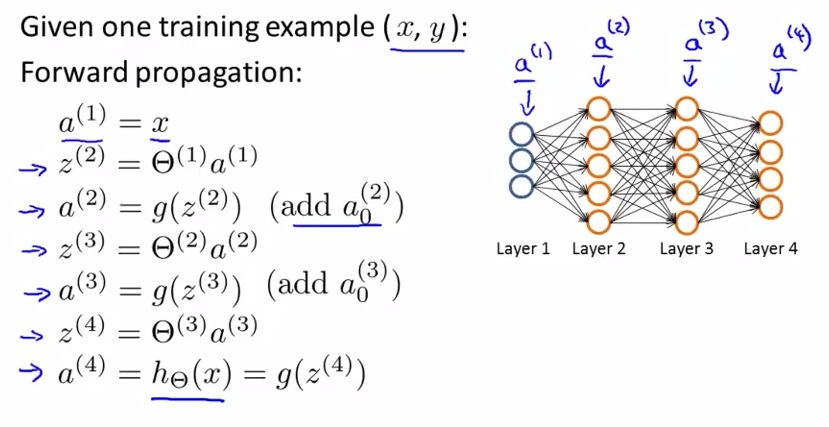• x,y only 1 training example, so just x and y
• add a0 as the biased term
• Next the partial derrivative will be calculated using Backpropagation algorithm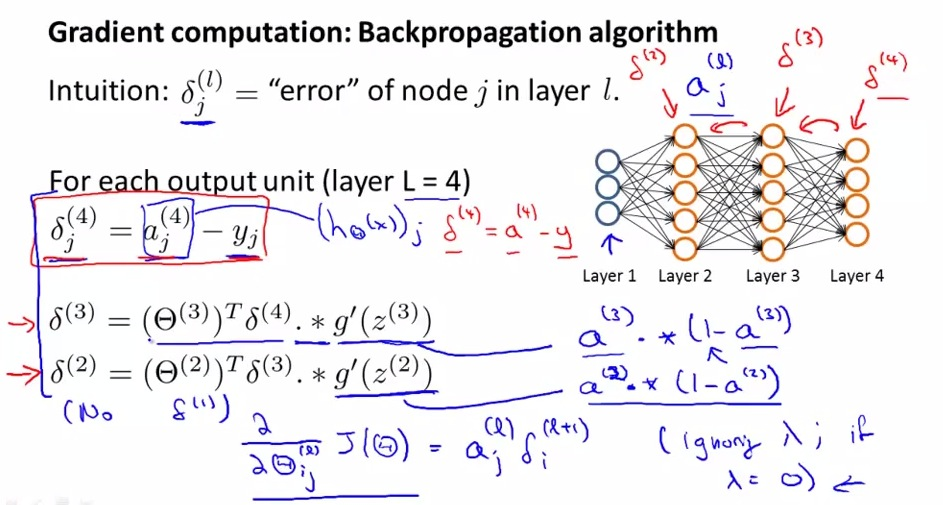• Each node for each layer will have error representation
• delta will capture the error for every node
• delta will be vector that has corresponding units with a and y
• a3 in blue printed is the activation layer in layer 3
• Backpropagation layer is propagating the error from last to first (reverse propagation)
• Next use backpropagation to minimize cost function with lots of training set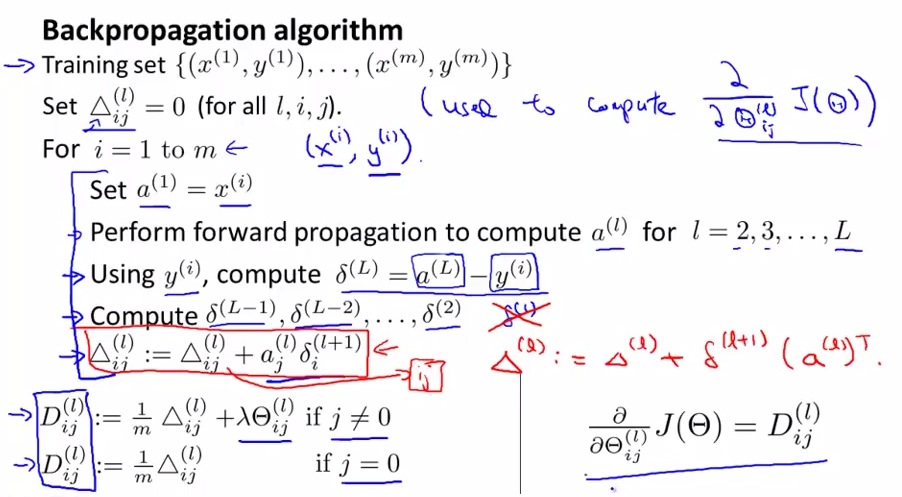• triangle is capital delta used to compute the partial derrivative
• error i not associated with input layer
• Finally, the formula shown above will calculate the minimized cost function used for gradient descent or advanced optimization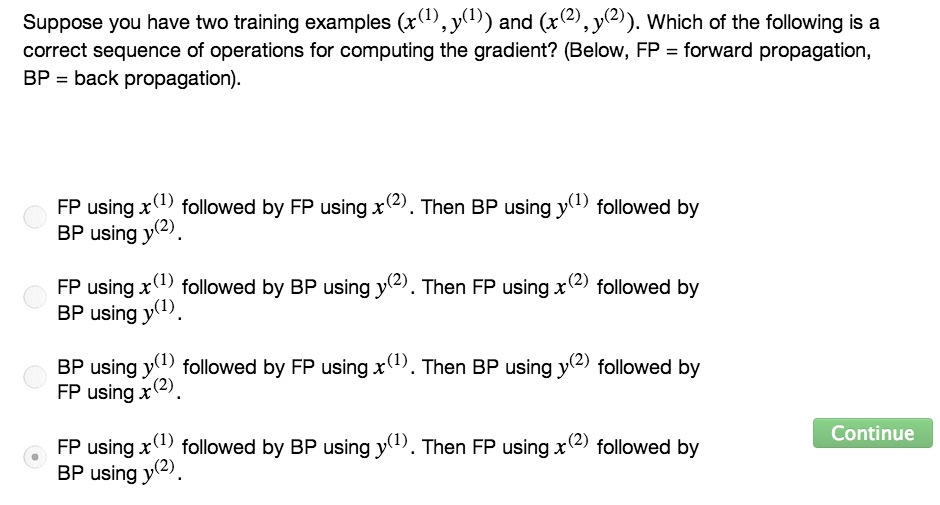• So this is the backpropagation algorithm that used to calculate the partial derrivative cost function (Neural Networks)used in gradien descent and advanced optimization